# Microeconomic Theory

## Quiz 6 : UncertaintyLooking for Economics Homework Help?

## Quiz 6 :Uncertainty

Question TypeGeorge is seen to place an even-money $100,000 bet on the Bulls to win the NBA Finals. If George has a logarithmic utility-of-wealth function and if his current wealth is$1,000,000, what must he believe is the minimum probability that the Bulls will win?
Free
Essay

The expected utility of betting when the probability of winning is p is given by below formula.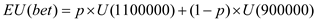The expected utility of not placing a bet is: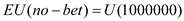The utility function is a logarithmic function. The expected utility of betting should be equal to expected utility of not betting.The above equation yields the value of p as.525, which is the minimum probability that the person placing the bet must believe that he would win.

TagsShow that if an individual's utility-of-wealth function is convex then he or she will prefer fair gambles to income certainty and may even be willing to accept somewhat unfair gambles. Do you believe this sort of risk-taking behavior is common? What factors might tend to limit its occurrence?
Free
Essay

It is required to be shown that a person whose utility curve is convex would prefer a fair gamble to certain income and may sometimes even prefer an unfair gamble.
A convex utility curve is shown in Figure 1.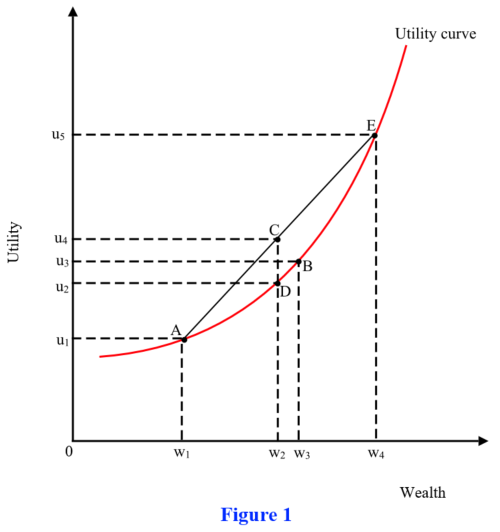If a gamble gives wealth w 1 and wealth w 4 with equal probability of 0.50, then the expected wealth under this gamble would be w 2 , as represented by the mid-point C of the line segment AE. Point C also shows that expected utility of this gamble is u 4.
If the person gets the wealth of w 2 with certainty, his utility would be u 2 , as represented by point D. Since this utility is lower than his expected utility under the gamble, he will prefer the gamble.
This shows that the person will prefer fair gamble to certain income.
The figure shows another point B. This point shows that if the person gets a wealth of w 3 with certainty, he gets a utility of u 3. Although wealth w 3 is higher than his expected wealth under the gamble, his utility from this wealth is still lower than his expected utility under the gamble. Therefore, the person may even prefer the gamble over a certain income that is even higher than the expected wealth under the gamble.
This shows that this person may even prefer unfair gambles.
Preferring unfair gambles is irrational behavior, but most real-life people do not exhibit such behavior. Most real-life people are risk averse. The case considered in this question is of a risk-loving person.

TagsAn individual purchases a dozen eggs and must take them home. Although making trips home is costless, there is a 50 percent chance that all the eggs carried on any one trip will be broken during the trip. The individual considers two strategies: (1) take all 12 eggs in one trip; or (2) take two trips with 6 eggs in each trip. a. List the possible outcomes of each strategy and the probabilities of these outcomes. Show that, on average, 6 eggs will remain unbroken after the trip home under either strategy. b. Develop a graph to show the utility obtainable under each strategy. Which strategy will be preferable? c. Could utility be improved further by taking more than two trips? How would this possibility be affected if additional trips were costly?
Free
Essay

a) In strategy 1, individual transports all eggs at once and there is a 50% chance that all eggs would break during a trip. So, there is a 50% chance that 12 eggs would be safely transported and a 50% chance that zero eggs would be left.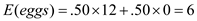In strategy 2, individual transports 6 eggs at once and there is 50% chance that all eggs would break during a trip. In trip 1, there is a 50% chance that 6 eggs would be safely transported and a 50% chance that zero eggs would be left. Similar logic applies to trip 2. Overall there is a 25% chance that all eggs would be safely transported and 25% chance that none would be.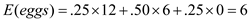b) The graph given below in Figure 1 shows the expected utility of different strategies. It is evident that the strategy 2 is preferable as its utility is higher.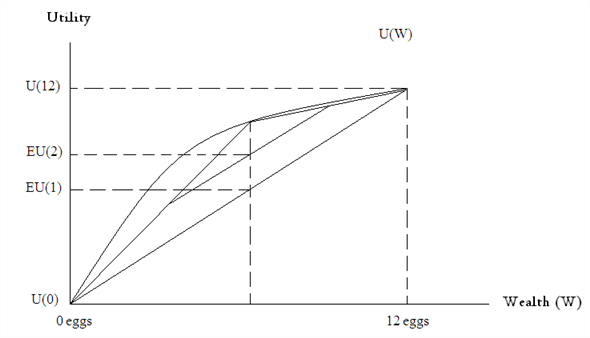Figure 1
c) The higher the number of trips, the higher the expected utility of that strategy. If one trip incurs a cost (c), then the possibility that more trips would give higher utility would be reduced. For example, if the second strategy requires one more trip like in the above example than the individual would prefer the second strategy only when: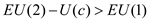TagsSuppose there is a 50-50 chance that a risk-averse individual with a current wealth of $20,000 will contract a debilitating disease and suffer a loss of$10,000. a. Calculate the cost of actuarially fair insurance in this situation and use a utility-of-wealth graph (such as shown in Figure 7.1) to show that the individual will prefer fair insurance against this loss to accepting the gamble uninsured. b. Suppose two types of insurance policies were available: (1) a fair policy covering the complete loss; and (2) a fair policy covering only half of any loss incurred. Calculate the cost of the second type of policy and show that the individual will generally regard it as inferior to the first. Reference: Figure 7.1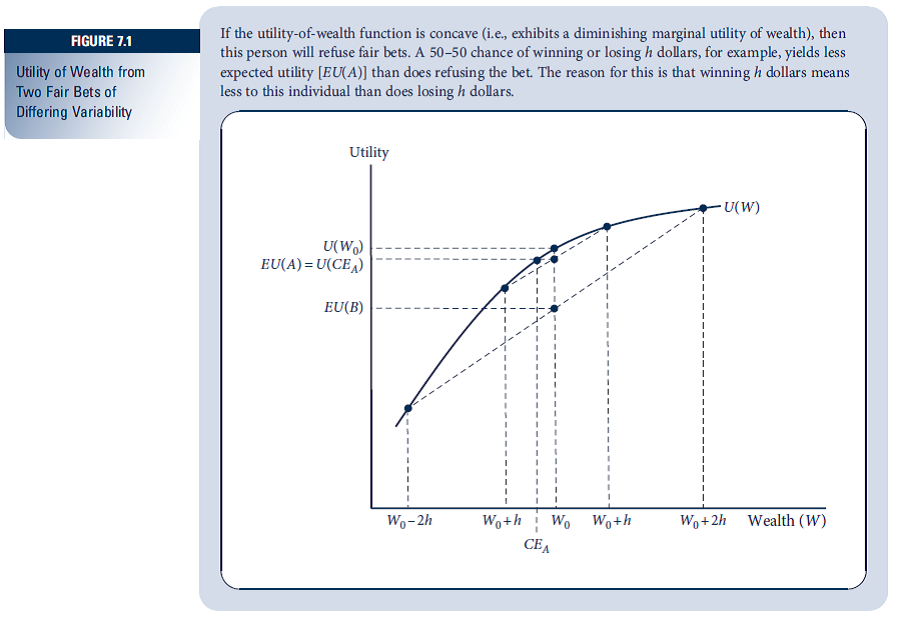Essay
TagsMs. Fogg is planning an around-the-world trip on which she plans to spend $10,000. The utility from the trip is a function of how much she actually spends on it (Y), given by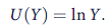a. If there is a 25 percent probability that Ms. Fogg will lose$1,000 of her cash on the trip, what is the trip's expected utility? b. Suppose that Ms. Fogg can buy insurance against losing the $1,000 (say, by purchasing traveler's checks) at an ''actuarially fair'' premium of$250. Show that her expected utility is higher if she purchases this insurance than if she faces the chance of losing the $1,000 without insurance. c. What is the maximum amount that Ms. Fogg would be willing to pay to insure her$1,000?
Essay
TagsIn deciding to park in an illegal place, any individual knows that the probability of getting a ticket is p and that the fine for receiving the ticket is f. Suppose that all individuals are risk averse (i.e., U '' (W) 0, where W is the individual's wealth). Will a proportional increase in the probability of being caught or a proportional increase in the fine be a more effective deterrent to illegal parking? Hint: Use the Taylor series approximation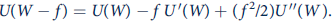Essay
TagsA farmer believes there is a 50-50 chance that the next growing season will be abnormally rainy. His expected utility function has the form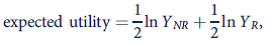where Y NR and Y R represent the farmer's income in the states of ''normal rain'' and ''rainy,'' respectively. a. Suppose the farmer must choose between two crops that promise the following income prospects: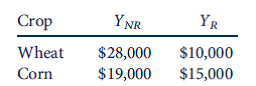Which of the crops will he plant? b. Suppose the farmer can plant half his field with each crop. Would he choose to do so? Explain your result. c. What mix of wheat and corn would provide maximum expected utility to this farmer? d. Would wheat crop insurance-which is available to farmers who grow only wheat and which costs $4,000 and pays off$8,000 in the event of a rainy growing season-cause this farmer to change what he plants?
Essay
TagsIn Equation 7.30 we showed that the amount an individual is willing to pay to avoid a fair gamble (h) is given by p = 0.5E( h 2 ) r ( W ), where r ( W ) is the measure of absolute risk aversion at this person's initial level of wealth. In this problem we look at the size of this payment as a function of the size of the risk faced and this person's level of wealth. a. Consider a fair gamble ( v ) of winning or losing $1. For this gamble, what is E ( v 2 )? b. Now consider varying the gamble in part (a) by multiplying each prize by a positive constant k. Let h = kv. What is the value of E ( h 2 )? c. Suppose this person has a logarithmic utility function U ( W ) = ln W. What is a general expression for r ( W )? d. Compute the risk premium ( p ) for k = 0.5, 1, and 2 and for W = 10 and 100. What do you conclude by comparing the six values? Reference: Equation 7.30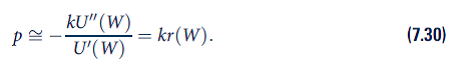Essay Answer: TagsReturn to Example 7.5, in which we computed the value of the real option provided by a flexible-fuel car. Continue to assume that the payoff from a fossil-fuel-burning car is A 1 (x) = 1 - x. Now assume that the payoff from the biofuel car is higher, A 2 (x) = 2x. As before, x is a random variable uniformly distributed between 0 and 1, capturing the relative availability of biofuels versus fossil fuels on the market over the future lifespan of the car. a. Assume the buyer is risk neutral with von Neumann-Morgenstern utility function U(x) = x. Compute the option value of a flexible-fuel car that allows the buyer to reproduce the payoff from either single-fuel car. b. Repeat the option value calculation for a risk-averse buyer with utility function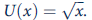c. Compare your answers with Example 7.5. Discuss how the increase in the value of the biofuel car affects the option value provided by the flexible-fuel car. Essay Answer: TagsHARA Utility The CARA and CRRA utility functions are both members of a more general class of utility functions called harmonic absolute risk aversion (HARA) functions. The general form for this function is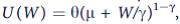where the various parameters obey the following restrictions: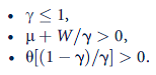The reasons for the first two restrictions are obvious; the third is required so that U ' 0. a. Calculate r (W) for this function. Show that the reciprocal of this expression is linear in W. This is the origin of the term harmonic in the function's name. b. Show that when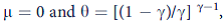, this function reduces to the CRRA function given in Chapter 7 (see footnote 17). c. Use your result from part (a) to show that if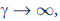, then r ( W ) is a constant for this function. d. Let the constant found in part (c) be represented by A. Show that the implied form for the utility function in this case is the CARA function given in Equation 7.35. e. Finally, show that a quadratic utility function can be generated from the HARA function simply by setting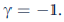. f. Despite the seeming generality of the HARA function, it still exhibits several limitations for the study of behavior in uncertain situations. Describe some of these shortcomings. Essay Answer: TagsProspect theory Two pioneers of the field of behavioral economics, Daniel Kahneman and Amos Tversky (winners of the Nobel Prize in economics in 2002), conducted an experiment in which they presented different groups of subjects with one of the following two scenarios: • Scenario 1: In addition to$1,000 up front, the subject must choose between two gambles. Gamble A offers an even chance of winning $1,000 or nothing. Gamble B provides$500 with certainty. • Scenario 2: In addition to $2,000 given up front, the subject must choose between two gambles. Gamble C offers an even chance of losing$1,000 or nothing. Gamble D results in the loss of \$500 with certainty. a. Suppose Standard Stan makes choices under uncertainty according to expected utility theory. If Stan is risk neutral, what choice would he make in each scenario? b. What choice would Stan make if he is risk averse? c. Kahneman and Tversky found 16 percent of subjects chose A in the first scenario and 68 percent chose C in the second scenario. Based on your preceding answers, explain why these findings are hard to reconcile with expected utility theory. d. Kahneman and Tversky proposed an alternative to expected utility theory, called prospect theory, to explain the experimental results. The theory is that people's current income level functions as an ''anchor point'' for them. They are risk averse over gains beyond this point but sensitive to small losses below this point. This sensitivity to small losses is the opposite of risk aversion: A risk-averse person suffers disproportionately more from a large than a small loss. (1) Prospect Pete makes choices under uncertainty according to prospect theory. What choices would he make in Kahneman and Tversky's experiment? Explain. (2) Draw a schematic diagram of a utility curve over money for Prospect Pete in the first scenario. Draw a utility curve for him in the second scenario. Can the same curve suffice for both scenarios, or must it shift? How do Pete's utility curves differ from the ones we are used to drawing for people like Standard Stan?
Essay
TagsMore on the CRRA function For the CRRA utility function (Equation 7.42), we showed that the degree of risk aversion is measured by 1 - R. In Chapter 3 we showed that the elasticity of substitution for the same function is given by 1/(1 - R). Hence the measures are reciprocals of each other. Using this result, discuss the following questions. a. Why is risk aversion related to an individual's willingness to substitute wealth between states of the world? What phenomenon is being captured by both concepts? b. How would you interpret the polar cases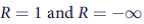in both the risk-aversion and substitution frameworks? c. A rise in the price of contingent claims in ''bad'' times (p b ) will induce substitution and income effects into the demands for W g and W b. If the individual has a fixed budget to devote to these two goods, how will choices among them be affected? Why might W g rise or fall depending on the degree of risk aversion exhibited by the individual? d. Suppose that empirical data suggest an individual requires an average return of 0.5 percent before being tempted to invest in an investment that has a 50-50 chance of gaining or losing 5 percent. That is, this person gets the same utility from W 0 as from an even bet on 1.055 W 0 and 0.955 W 0. (1) What value of R is consistent with this behavior? (2) How much average return would this person require to accept a 50-50 chance of gaining or losing 10 percent? Note: This part requires solving nonlinear equations, so approximate solutions will suffice. The comparison of the risk- reward trade-off illustrates what is called the equity premium puzzle in that risky investments seem actually to earn much more than is consistent with the degree of risk aversion suggested by other data. See N. R. Kocherlakota, ''The Equity Premium: It's Still a Puzzle,'' Journal of Economic Literature (March 1996): 42-71
EssayGraphing risky investments Investment in risky assets can be examined in the state-preference framework by assuming that W * dollars invested in an asset with a certain return r will yield W * (1 + r) in both states of the world, whereas investment in a risky asset will yield W * (1 + r g ) in good times and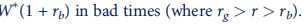a. Graph the outcomes from the two investments. b. Show how a ''mixed portfolio'' containing both risk-free and risky assets could be illustrated in your graph. How would you show the fraction of wealth invested in the risky asset? c. Show how individuals' attitudes toward risk will determine the mix of risk-free and risky assets they will hold. In what case would a person hold no risky assets? d. If an individual's utility takes the constant relative risk aversion form (Equation 7.42), explain why this person will not change the fraction of risky assets held as his or her wealth increases.The portfolio problem with a Normally distributed risky asset In Example 7.3 we showed that a person with a CARA utility function who faces a Normally distributed risk will have expected utility of the form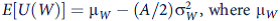is the expected value of wealth and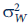is its variance. Use this fact to solve for the optimal portfolio allocation for a person with a CARA utility function who must invest k of his or her wealth in a Normally distributed risky asset whose expected return is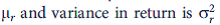(your answer should depend on A). Explain your results intuitively